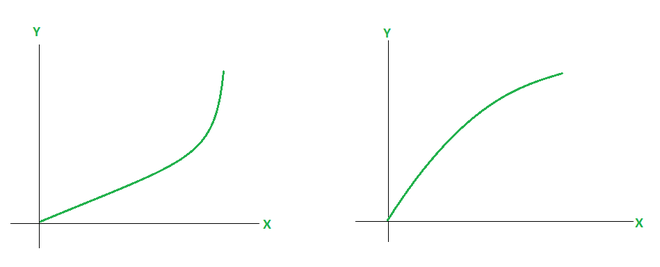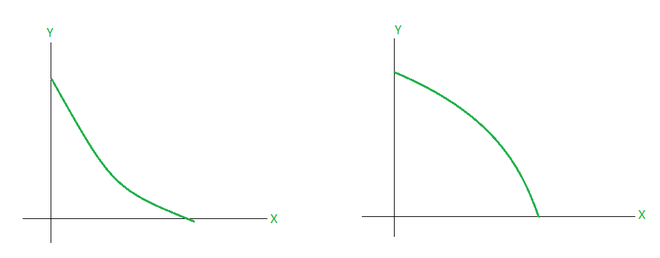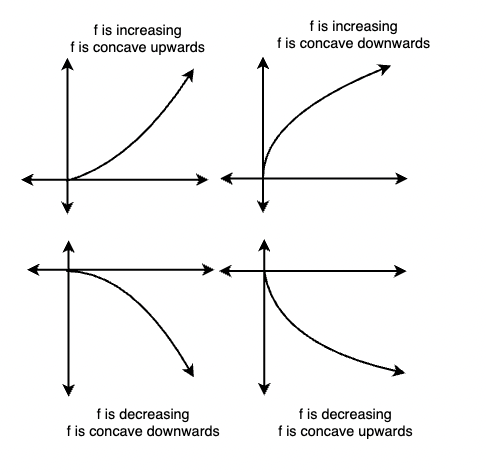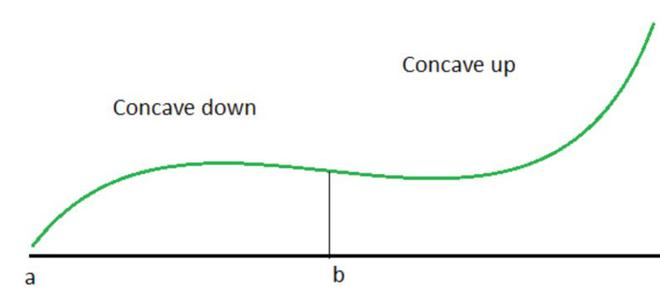Related Articles

# Inflection Points

• Last Updated : 02 Jun, 2021

Consider the two functions given in the figure below, while both of these functions look similar.  What differentiates them is their bend, notice that one of them looks opening upwards and the other looks closing downwards. This trend is called concavity, the first figure is concave upwards and the second one is concave downwards. Looking for these trends helps us to generate the curves for the functions.### Concavity

A function is said to be concave up on an interval if the graph of that function lies above any tangent that is drawn on any point of the interval. Similarly, the function is said to be concave downwards if the graph of the function lies below any tangent that is drawn on any point of the interval. Intuitively, concavity related to the rate of the increase. It is known that derivatives give us the rate of change, which means they tell us if the function is increasing or decreasing. Concavity is related to the rate of change of the rate of change, that is it must be related to double derivatives.

In the figure shown above, the first function increases at an increasing rate while the second function increases at a decreasing rate. It is also evident from the fact that in the first function, the tangents become steeper and steeper and vice versa for the second function. The figure below shows a similar scenario but with decreasing functions.To summarize these observations, the figure below shows a consolidated comparison of all these different cases.To formalize the above observations,

Concavity Test:

Consider a function f(x) which is differentiable and has derivatives and double derivatives as f’ and f” on the interval I.

1. f(x) is considered concave up if f”(x) > 0 for all the points in the interval I.
2. (x) is considered concave down if f”(x) < 0 for all the points in the interval I.

### Inflection Points

Sometimes in function trajectories, the function goes from concave upwards to concave downwards or vice versa. Now when the function goes through such changes, there is a certain point that works as a boundary. This means if before this point the function was concave up, after this point the function will be opposite of that. This point is called the point of inflection. The figure shows this point.Geometrically speaking, the tangent drawn at this point is neither above the graph nor below the graph. The tangent intersects the function’s graph at this point.

Point of inflection is the point where function goes from being concave upwards to concave downwards and vice-versa. Let’s say f(x) is the function which has a point x = c as it’s inflection point. Then,

f”(c) = 0 or f”(c) does not exist.

Notice that when f”(c) = 0, it means that basically x = c is a critical point of f'(x) = 0. So, in this scenario, three cases are possible:

1. Case (i): The sign of f”(x) changed from positive to negative at the inflection point. This means that the function went from being concave upwards to being concave downwards.
2. Case (ii): The sign of f”(x) changed from negative to positive at the inflection point. This means that the function went from being concave downwards to being concave upwards.
3. Case (iii): The sign is not changing, which means the double derivative is already zero throughout the function. In this case, the inflection point won’t be there.

These three cases show that it is not necessary that if the double derivative is zero at a point, it is an inflection point.

Inflection Point Test

Consider a function f(x), to determine the inflection points.

1. Find f”(x).
2. Solve the equation f”(x) = 0 and find all the critical points of f'(x).
3. Use the number line to see at which points the double derivative changes sign.

Let’s see some sample problems with these concepts.

### Sample Problems

Question 1: Find out the concavity of the given function between the interval [0,1].

f(x) = x2 + 4

Solution:

To check for the concavity, let’s look at the second derivative of the function..

f(x) = x2 + 4

Differentiating w.r.t x,

f'(x) = 2x

Differentiating w.r.t x again,

f”(x) = 2 > 0

This is greater than zero, the function is concave upwards in the given interval.

Question 2: Find out the concavity of the given function between the interval [2,4].

f(x) = x3 + 4

Solution:

To check for the concavity, let’s look at the second derivative of the function..

f(x) = x3

Differentiating w.r.t x,

f'(x) = 3x2

Differentiating w.r.t x again,

f”(x) = 6x > 0

This is greater than zero, the function is concave upwards in the given interval.

Question 3: Find out the point of inflection for the given function.

f(x) = x3

Solution:

To check for the point of inflection., let’s look at the second derivative of the function..

f(x) = x3 + 4

Differentiating w.r.t x,

f'(x) = 3x2

Differentiating w.r.t x again,

f”(x) = 6x = 0

Solving f”(x) = 0, it shows that x = 0 is the critical point of f'(x).

Now let’s check the value of f”(x) before and after the critical point.

for x < 0, f”(x) < 0.

for x > 0, f”(x) > 0.

Thus, the function changes from concave downward to concave upward. Thus, x = 0 is the inflection point.

Question 4: Find out the point of inflection for the given function.

f(x) = x3 + x2 + 3

Solution:

To check for the point of inflection., let’s look at the second derivative of the function..

f(x) = x3 + x2 + 3

Differentiating w.r.t x,

f'(x) = 3x2 + 2x

Differentiating w.r.t x again,

f”(x) = 6x + 2 = 0

Solving f”(x) = 0, it shows that x =is the critical point of f'(x).

Now let’s check the value of f”(x) before and after the critical point.

for x < -1, f”(x) < 0.

for x > 0, f”(x) > 0.

Thus, the function changes from concave downward to concave upward. Thus, x =is the inflection point.

Question 5: Find out the point of inflection for the given function.

f(x) = x4 – 2x2 + 3x

Solution:

To check for the point of inflection., let’s look at the second derivative of the function..

f(x) = x4 – 2x2 + 3x

Differentiating w.r.t x,

f'(x) = 4x3 – 4x + 3

Differentiating w.r.t x again,

f”(x) = 12x2 – 4x + 3= 0

Solving f”(x) = 0, it shows that x =is the critical point of f'(x).

Now let’s check the value of f”(x) before and after the critical point.

for x < -1, f”(x) < 0.

for x > 0, f”(x) > 0.

Thus, the function changes from concave downward to concave upward. Thus, x =is the inflection point.

Question 6: Find out the point of inflection for the given function for the interval [0,1].

f(x) =Solution:

To check for the point of inflection., let’s look at the second derivative of the function..

f(x) =Differentiating w.r.t x,

f'(x) =⇒ f'(x) =Differentiating w.r.t x again,

f”(x) =Solving f”(x) = 0 for the given interval. There is no solution to this equation in the given interval.

Thus, inflection point does not exist in [0,1].

Attention reader! All those who say programming isn’t for kids, just haven’t met the right mentors yet. Join the  Demo Class for First Step to Coding Coursespecifically designed for students of class 8 to 12.

The students will get to learn more about the world of programming in these free classes which will definitely help them in making a wise career choice in the future.

My Personal Notes arrow_drop_up